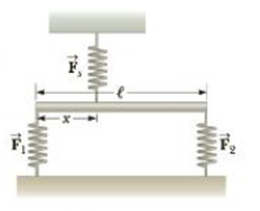Chapter 8, Problem 83AP

Chapter
Section
Textbook Problem

A 2.35-kg uniform bar of length ℓ = 1.30 m is held in a horizontal position by three vertical springs as in Figure P8.83. The two lower springs are compressed and exert upward forces on the bar of magnitude F1 = 6.80 N and F2 = 9.50 N, respectively. Find (a) the force Fs exerted by the top spring on the bar, and (b) the location x of the upper spring that will keep the bar in equilibrium.Figure P8.83

(a)

To determine
The force exerted by the top spring.

Explanation

Given Info:

The forces exerted by the lower springs are 6.80N and 9.50N

The length of the uniform bar is 1.30m

The mass of the bar is 2.35kg

Figure below shows the restoring forces acting on the spring and the gravitational force acting on the bar.

The upper spring is stretched. Hence, the restoring force acting on it upward direction and the weight of the bar acts downward from the its centre of gravity.

Since the whole system is in equilibrium, the condition for equilibrium is,

Fs+F1+F2mg=0

Substitute 6.80N for F1 , 9.50N for F2 , 2

(b)

To determine
The location x of the upper spring.

Still sussing out bartleby?

Check out a sample textbook solution.

See a sample solution

The Solution to Your Study Problems

Bartleby provides explanations to thousands of textbook problems written by our experts, many with advanced degrees!

Get Started

Find more solutions based on key concepts